# Choosability of Graph Powers

 Importance: Medium ✭✭
 Author(s): Noel, Jonathan A.
 Subject: Graph Theory » Coloring » » Vertex coloring
 Keywords: choosability chromatic number list coloring square of a graph
 Posted by: Jon Noel on: July 13th, 2013
Question  (Noel, 2013)   Does there exist a function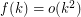such that for every graph,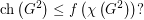For a survey of choosability, including relevant definitions, see [Noe] or click here.

The List Square Colouring Conjecture, due to Kostochka and Woodall [KW], states that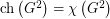for every graph. This was disproved by Kim and Park [KP], who proved that there is a sequence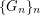of graphs and a constant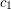such that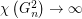andfor all. To obtain this lower bound from the construction of Kim and Park, one can apply the well-known result of Alon [Alo].

It may be the case that the correct upper bound for all graphs is of the same order of magnitude as the example in the result of Kim and Park.

Question  (Noel, 2013)   Does there exist a positive constant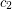such that every graphsatisfies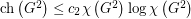?

By calculating the clique number and maximum degree of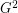, one can easily show that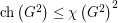(this observation is due to Young Soo Kwon), but it seems that no significantly better bound is known.

Proposition   Ifcontains an edge, then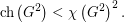Proof   We observe the following bounds: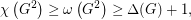Therefore, since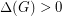, we haveThis completes the proof.

These questions are related to a problem of Zhu (see Doug West's webpage for more info) who asked whether there exists an integersuch that for every graph, we have thathas choice number equal to chromatic number. This conjecture has been disproved independently by Kim, Kwon and Park [KKP] and Kosar, Petrickova, Reigniger and Yeager [KPRY]. The example of [KPRY] also yields, for every, a sequenceof graphs and a constantsuch thatandfor all. They ask the following, more general, questions:

Question  (Kosar et al., 2013)   Given, does there exist a function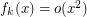such that for every graph,To our knowledge, it is not known whether there exists a function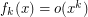such that the same conclusion holds. (Intuitively, it seems that higher values ofshould yield a smaller separation between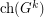and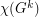; however, there seems to be no hard evidence to support this.)

Question  (Kosar et al., 2013)   Given, does there exist a positive constantsuch that every graphsatisfies? Moreover, can the constantbe made independent of?

These questions are also related to the so-called List Total Colouring Conjecture of Borodin, Kostochka and Woodall [BKW], which says that the total graph of a multigraph always satisfies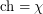. Given a multigraph, the total graph ofcan be obtained by subdividing every edge ofand then taking the square of the resulting graph.

## Bibliography

[Alo] Noga Alon. Choice numbers of graphs: a probabilistic approach. Combin. Probab. Comput., 1(2):107–114, 1992.

[BKW] Oleg V. Borodin, Alexandr V. Kostochka, and Douglas R. Woodall. List edge and list total colourings of multigraphs. J. Combin. Theory Ser. B, 71(2):184–204, 1997.

[KP] Seog-Jin Kim and Boram Park: Counterexamples to the List Square Coloring Conjecture, submitted.

[KKP] Seog-Jin Kim, Young Soo Kwon and Boram Park: Chromatic-choosability of the power of graphs.

[KPRY] Nicholas Kosar, Sarka Petrickova, Benjamin Reiniger, Elyse Yeager: A note on list-coloring powers of graphs.

[KW] Alexandr V. Kostochka and Douglas R. Woodall. Choosability conjectures and multicircuits, Discrete Math., 240 (2001), 123--143.

[Noe] Jonathan A. Noel. Choosability of Graphs with Bounded Order: Ohba's Conjecture and Beyond, Master's thesis. McGill University (2013). pdf.

* indicates original appearance(s) of problem.